Related Articles
Class 9 NCERT Solutions- Chapter 10 Circles – Exercise 10.4
• Last Updated : 03 Jan, 2021

### Question 1. Two circles of radii 5cm and 3cm intersect at two points and the distance between their centers is 4 cm. Find the length of the common chord.

Solution:

Given: OP=4cm, AP=3cm, QR=5cm

To find: In ∆APO:

AO²=5²=25

OP²=4²=16

AP²=3²=9

OP²+AP²=AO²

BY converse of Pythagoras theorem

ΔAPO: is a right ∠D=P

Now, in the bigger circle OP is perpendicular AB

AP=½AB          —————-(perpendicular from the center of circle  to a chord bisect the chord )

3=½AB

6=AB

Therefore the length of common chord is 6cm.### Question 2. If two equal chords of a circle intersect within the circle, prove that the line joining the point of intersection to the center makes equal angles with the chords.

Solution:

Given: Equal chord AB & CD intersect at P.

To find: AP=PD and PB=PC

Construction: Draw OM perpendicular AB ,ON perpendicular CD and join OP.

Because perpendicular from center bisect the chord

AM=MB=½AB     also   CN=ND=½CD

AM=MB=CN=ND        ——————1

Now, In ∆OMP  and  ∆ONP

ANGLE M=ANGLE N              [90° both]

OP=OP               [COMMON]

ON=OM              [equal chords are equilateral from center]

∆OMP≅∆ONP

Therefore MP=PN           (C.P.C.T.)      ——————2

i)from   1 and 2

AM+MP=ND+AN

AP=PD

ii)MB-MP=CN=PN

PB=PC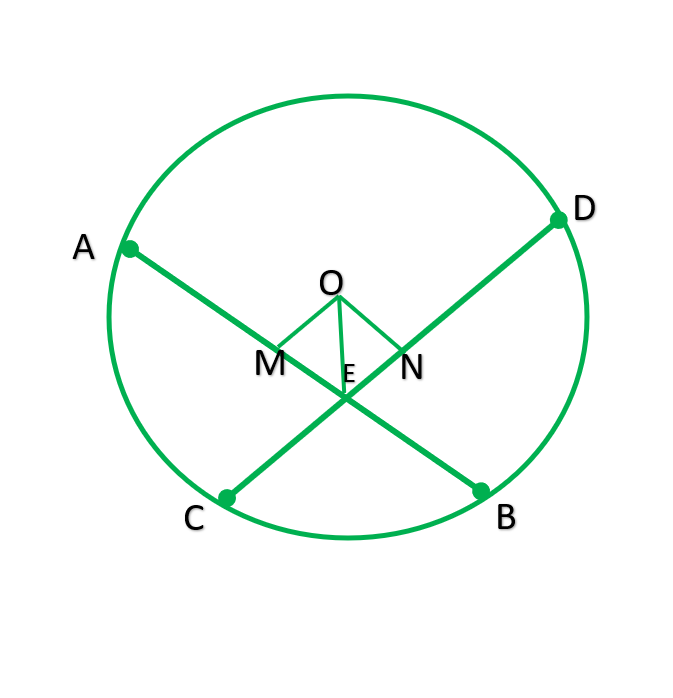### Question 3. If two equal chords of a circle intersect within the circle, prove that the line joining the point of intersection to the Centre makes equal angles with the chords.

Solution:

Given: Equal chords AB and CD intersect at P.

To prove: angle1=angle=2

Construction: Draw OM perpendicular AB & ON perpendicular CD.

Solution: In ∆OMP & ∆ONP

Angle M= Angel N      [90 °  each]

OP=OP           [common]

OM=ON      —————[ Equal chords are equal distant from center]

∆OMP≅∆ONP  ———-[R.H.S]

∠1=∠2     ———–[C.P.C.T]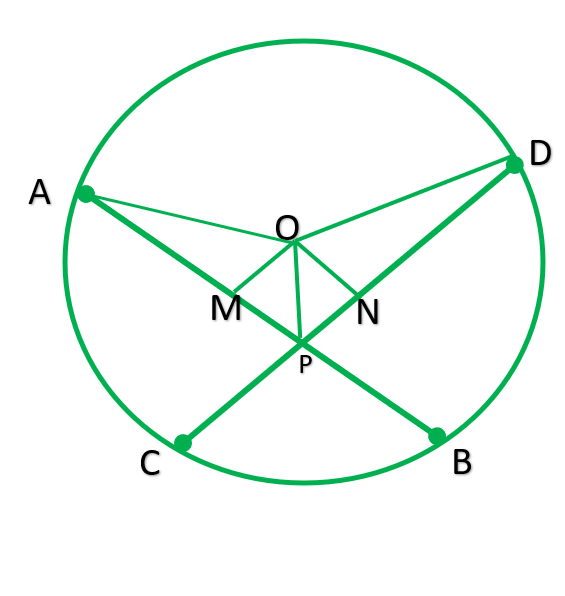### Question 4. If a line intersects two concentric circles (circles with the same centre) with centre O at A, B, C and D, prove that AB = CD (see Figure).

Solution:

Given : two concentric circle with  O. A line intersect them at A, B, C , and  D

To prove: AB=CD

construction:  Draw OM ⊥ AD ,In bigger circle AD is chord OM  ⊥ AD.

∴AM=MD              —————-[⊥ from center of circle of a circle bisects  the chord]         __________    1

The smaller circle :

BC is chord  OM ⊥ BC

BM=MC         ——————-[⊥ from center of circle of a circle bisects  the chord]             __________    2

subtracting  1-2

AM-BM=MD-MC

AB=CD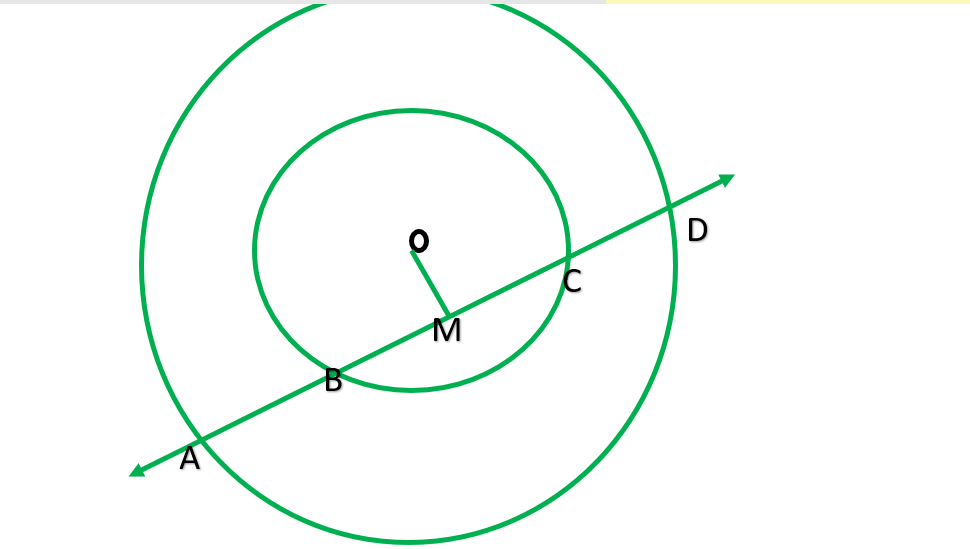### Question 5. Three girls Reshma, Salma and Mandip are playing a game by standing on a circle of radius 5m drawn in a park. Reshma throws a ball to Salma, Salma to Mandip, Mandip to Reshma. If the distance between Reshma and Salma and between Salma and Mandip is 6m each, what is the distance between Reshma and Mandip?

Solution:

To find RM=?

Let Reshma, Salma and Mandip be R,S,M

Construction: Draw OP ⊥ RS join OR and OS.

RP=½RS   ___________[⊥ from center bisects the chord]

RP=½*6=3m

In right ΔORP

OP²=OR²- PR²

OP= √ 5² -3²

=√259    =√16      =4

Area of ΔORS=½*RS*OP

=½*6*4=12m²     —————–1

Now, ∠N=90°

Area of ΔORS=½*SO*RN

=½*SO*RN     ——————-2

Above ,1=2

12=½*5*RN

12/5*2=RN

RN=4.8

RM=2*RN _________________[⊥ from center bisects the chord]

=2*4.8

9.6m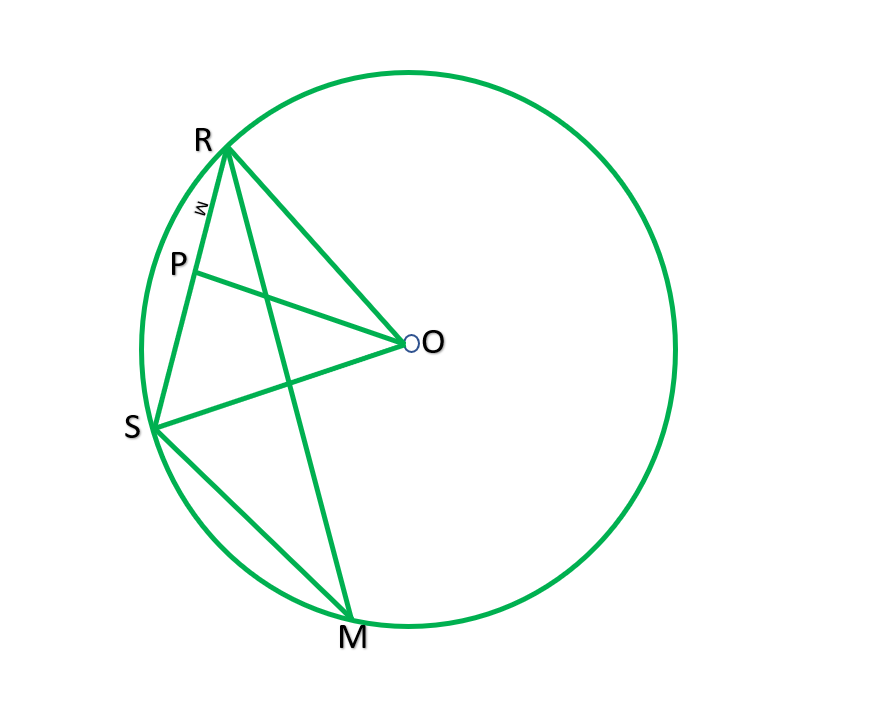### Question 6. A circular park of radius 20m is situated in a colony. Three boys Ankur, Syed and David are sitting at equal distance on its boundary. Each boy has toy telephone in his hand to talk with each other. Find the length of the string of each phone.

Solution:

Draw AM⊥SD

AS=SD=AD

ASD is the equilateral  Δ

Let each side of Δ-2xm

SM=2x/2=x

Now in Δ DMS, by the Pythagoras theorem

AM²+SM²=AS²

AM²= AS²- SM²

AM=√(2x²+x² )

==√(3x² )

AM =√3x

OM=AM-AO

OM=√3x-20

Now in right ΔOMS

OM²+SM²=SO²

(√3x-20)²+2x²+x²=20²

20²+400-40√3x+x^2=400

4x²=40√3x

4xx=40√3x

X=(40√3)/4

X=10√3x

Length of each string =2x

=2*10√3xm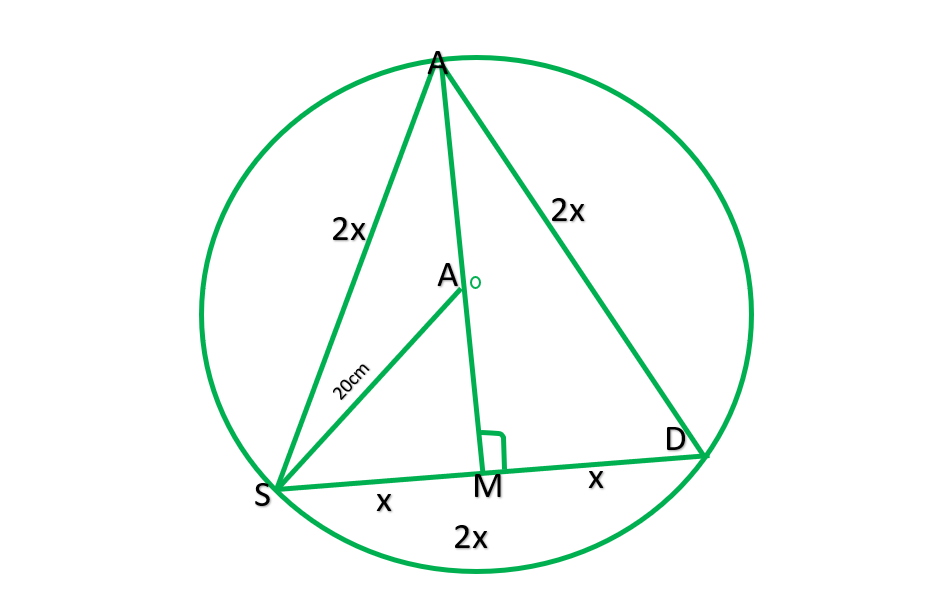Attention reader! Don’t stop learning now. Get hold of all the important DSA concepts with the DSA Self Paced Course at a student-friendly price and become industry ready.

My Personal Notes arrow_drop_up